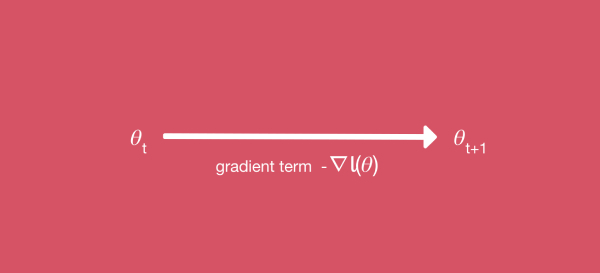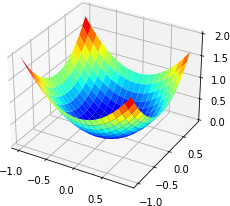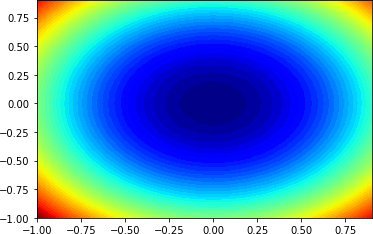• +91-9872993883
• +91-8283824812
• info@ris-ai.com

# Gradient Descent With Nesterov Momentum ¶

## Gradient Descent: An Introduction to 1 of Machine Learning’s Most Popular Algorithms

Gradient descent is an optimization algorithm. this works is you define a loss function l(θ) that expresses how well your weights & biases θ allow the network to fit your training data. A higher loss means that the network is bad and makes a lot of errors while a low loss generally means that the network performs well. You can then train your network by adjusting the network parameters in a way that reduces the loss.### Gradient Descent with Nesterov Momentum ¶

Nesterov momentum is a simple change to normal momentum. Here the gradient term is not computed from the current position θ(t) in parameter space but instead from a position θ(intermediate)=θ(t)+μv(t). This helps because while the gradient term always points in the right direction , the momentum term may not. If the momentum term points in the wrong direction or overshoots, the gradient can still "go back" and correct it in the same update step.##### Gradient Descent Optimization Algorithm with Nesterov Momentum ¶

First an optimization function.

We will use a simple two-dimensional function that squares the input of each dimension and define the range of valid inputs from -1.0 to 1.0.

We can create a three-dimensional plot of the dataset to get a feeling for the curvature of the response surface.

In :
# 3d plot of the test function
from numpy import arange
from numpy import meshgrid
from matplotlib import pyplot

# objective function
def objective(x, y):
return x**2.0 + y**2.0

# define range for input
r_min, r_max = -1.0, 1.0
# sample input range uniformly at 0.1 increments
xaxis = arange(r_min, r_max, 0.1)
yaxis = arange(r_min, r_max, 0.1)
# create a mesh from the axis
x, y = meshgrid(xaxis, yaxis)
# compute targets
results = objective(x, y)
# create a surface plot with the jet color scheme
figure = pyplot.figure()
axis = figure.gca(projection='3d')
axis.plot_surface(x, y, results, cmap='jet')
# show the plot
pyplot.show()this creates a two-dimensional contour plot of the objective function.

We can see the bowl shape compressed to contours shown with a color gradient. We will use this plot to plot the specific points explored during the progress of the search.

In :
# contour plot of the test function
from numpy import asarray
from numpy import arange
from numpy import meshgrid
from matplotlib import pyplot

# objective function
def objective(x, y):
return x**2.0 + y**2.0

# define range for input
bounds = asarray([[-1.0, 1.0], [-1.0, 1.0]])
# sample input range uniformly at 0.1 increments
xaxis = arange(bounds[0,0], bounds[0,1], 0.1)
yaxis = arange(bounds[1,0], bounds[1,1], 0.1)
# create a mesh from the axis
x, y = meshgrid(xaxis, yaxis)
# compute targets
results = objective(x, y)
# create a filled contour plot with 50 levels and jet color scheme
pyplot.contourf(x, y, results, levels=50, cmap='jet')
# show the plot
pyplot.show()### Gradient Descent Optimization With Nesterov Momentum ¶

nesterov() that takes the names of the objective function and the derivative function, an array with the bounds of the domain and hyperparameter values for the total number of algorithm iterations, the learning rate, and the momentum, and returns the final solution and its evaluation

In :
# gradient descent optimization with nesterov momentum for a two-dimensional test function
from math import sqrt
from numpy import asarray
from numpy.random import rand
from numpy.random import seed

# objective function
def objective(x, y):
return x**2.0 + y**2.0

# derivative of objective function
def derivative(x, y):
return asarray([x * 2.0, y * 2.0])

# gradient descent algorithm with nesterov momentum
def nesterov(objective, derivative, bounds, n_iter, step_size, momentum):
# generate an initial point
solution = bounds[:, 0] + rand(len(bounds)) * (bounds[:, 1] - bounds[:, 0])
# list of changes made to each variable
change = [0.0 for _ in range(bounds.shape)]
for it in range(n_iter):
# calculate the projected solution
projected = [solution[i] + momentum * change[i] for i in range(solution.shape)]
# calculate the gradient for the projection
# build a solution one variable at a time
new_solution = list()
for i in range(solution.shape):
# calculate the change
change[i] = (momentum * change[i]) - step_size * gradient[i]
# calculate the new position in this variable
value = solution[i] + change[i]
# store this variable
new_solution.append(value)
# evaluate candidate point
solution = asarray(new_solution)
solution_eval = objective(solution, solution)
# report progress
print('>%d f(%s) = %.5f' % (it, solution, solution_eval))
return [solution, solution_eval]

# seed the pseudo random number generator
seed(1)
# define range for input
bounds = asarray([[-1.0, 1.0], [-1.0, 1.0]])
# define the total iterations
n_iter = 30
# define the step size
step_size = 0.1
# define momentum
momentum = 0.3
# perform the gradient descent search with nesterov momentum
best, score = nesterov(objective, derivative, bounds, n_iter, step_size, momentum)
print('Done!')
print('f(%s) = %f' % (best, score))

>0 f([-0.13276479  0.35251919]) = 0.14190
>1 f([-0.09824595  0.2608642 ]) = 0.07770
>2 f([-0.07031223  0.18669416]) = 0.03980
>3 f([-0.0495457   0.13155452]) = 0.01976
>4 f([-0.03465259  0.0920101 ]) = 0.00967
>5 f([-0.02414772  0.06411742]) = 0.00469
>6 f([-0.01679701  0.04459969]) = 0.00227
>7 f([-0.01167344  0.0309955 ]) = 0.00110
>8 f([-0.00810909  0.02153139]) = 0.00053
>9 f([-0.00563183  0.01495373]) = 0.00026
>10 f([-0.00391092  0.01038434]) = 0.00012
>11 f([-0.00271572  0.00721082]) = 0.00006
>12 f([-0.00188573  0.00500701]) = 0.00003
>13 f([-0.00130938  0.0034767 ]) = 0.00001
>14 f([-0.00090918  0.00241408]) = 0.00001
>15 f([-0.0006313   0.00167624]) = 0.00000
>16 f([-0.00043835  0.00116391]) = 0.00000
>17 f([-0.00030437  0.00080817]) = 0.00000
>18 f([-0.00021134  0.00056116]) = 0.00000
>19 f([-0.00014675  0.00038964]) = 0.00000
>20 f([-0.00010189  0.00027055]) = 0.00000
>21 f([-7.07505806e-05  1.87858067e-04]) = 0.00000
>22 f([-4.91260884e-05  1.30440372e-04]) = 0.00000
>23 f([-3.41109926e-05  9.05720503e-05]) = 0.00000
>24 f([-2.36851711e-05  6.28892431e-05]) = 0.00000
>25 f([-1.64459397e-05  4.36675208e-05]) = 0.00000
>26 f([-1.14193362e-05  3.03208033e-05]) = 0.00000
>27 f([-7.92908415e-06  2.10534304e-05]) = 0.00000
>28 f([-5.50560682e-06  1.46185748e-05]) = 0.00000
>29 f([-3.82285090e-06  1.01504945e-05]) = 0.00000
Done!
f([-3.82285090e-06  1.01504945e-05]) = 0.000000

In [ ]: